﻿ Gregory Chalenko - Compositing Artist and TD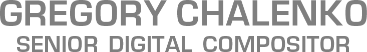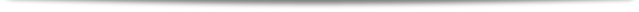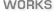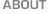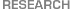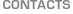The Math Behind the Apply Modes

Understanding of the math used for combining two images in the Merge tool lets you work in a more efficient way, getting predictable results straight away instead of experimenting with combinations of parameters. This knowledge is also important to fix possible problems quickly yet elegantly.

## Normal

In this mode, the Merge offers an extra Subtractive/Additive control which lets you select between additive and subtractive compositing or even make a mix between these types.
The two formulas of the Normal mode are:
 Result Color = Background Color ∗ (1 − Foreground Alpha ∗ Alpha Gain ∗ (1 − Burn In)) + Foreground Color - for additive Result Color = Background Color ∗ (1 − Foreground Alpha ∗ Alpha Gain ∗ (1 − Burn In)) + Foreground Color ∗ Foreground Alpha ∗ Alpha Gain - for subtractive
As you can see, the difference between the additive and subtractive formulas is that in the additive one, the foreground doesn't get multiplied by its Alpha.
This is because additive compositing implies that your images are already pre-multiplied by Alpha. It normally means that completely transparent areas are black and 0 in Alpha corresponds to 0, 0, 0 in R, G, B.
The additive approach is used in 3D and professional compositing software.

The subtractive approach is mostly used in editing software, Adobe-ware and other programs working in low color depth. It works with "un-premultiplied", or pre-divided by Alpha images (depending on the way the Alpha was created).
In such images the color extends beyond object edges and in semi-transparent areas it has the same values as in completely opaque ones. The multiplication by Alpha is performed on the fly at the time of putting the layers together, as you can see from the subtractive formula.
A benefit of this workflow is that all the color tools operate the values unaffected by transparency (straight colors), so highlights remain highlights and shadows remain shadows regardless of how transparent or blurred the object is.
In software like Fusion, which is capable of processing the images in 32 bit per channel mode, it's easily fixed with pre-dividing by Alpha (sometimes strangely called un-premultiplying), but in 8-bit or 16 bit integer mode you will experience color distortions after performing sequential divisions and multiplications due to rounding losses.

Fusion supports both modes as it's capable of working in multiple color depth.

As you can conclude from the Additive formula of the Normal mode, if you set the Alpha Gain = 0 in the Merge and leave the Subtractive/Additive = 1 by default, you will get a pure addition of the Background and Foreground color. The Foreground Alpha is completely ignored in this case.

Here is what happening if we substitute Alpha Gain by 0 in the formula:

 Result Color = Background Color ∗ (1 − Foreground Alpha ∗ Alpha Gain ∗ (1 − Burn In)) + Foreground Color ↙ Result Color = Background Color ∗ (1 − Foreground Alpha ∗ 0 ∗ (1 − Burn In)) + Foreground Color ↙ Result Color = Background Color ∗ (1 − 0) + Foreground Color

If you set Burn In = 1, the resulting color will be a pure addition too, but the Foreground Alpha will be also added to the Background Alpha. Most of the time it's undesirable, because adding two images with Alpha together, you will get Alpha > 1 (Alpha = 2 in completely opaque areas).

But there is a case when such handling of the alpha is preferable. Imagine, that you separate your image onto two layers by cutting a part of it with a mask to process it separately. If you merge it back over the original in the Normal mode, you will get a semi-transparent border of the mask in the resulting image. This is because in the default Normal mode you first multiply the Background Color with inverted Foreground Alpha when you cut a part of the image and then do it again while pasting it on top.

Why not to leave the background layer intact while cutting a part of it as a foreground? Good question - simple answer: semi-transparent areas will reduce transparency twice and mix the original color with altered one.

## Multiply

Result Color = Background ColorForeground Color

This mode is used to composite shadows, apply lighting maps and masks.
In the areas where the Foreground Color is 1, the Background Color remains unaffected. If the Foreground Color is 0, the result is black.

## Screen

Result Color = Foreground Color + Background ColorForeground ColorBackground Color
or
Result Color = 1 − (1Background Color) ∗ (1Foreground Color)

Screen is essentially, inverted Multiply. You can clearly see it from the second version of the formula, where the colors of both layers get inverted and then multiplied together.
This mode is used, for example, to combine reflection and transparency on the glass.
The result is never more than 1.

Here is an example of how exactly the formulas of the modes described above and some others work:
ApplyModes.comp© 07.09.2010 - 23.01.2015 Gregory Chalenko